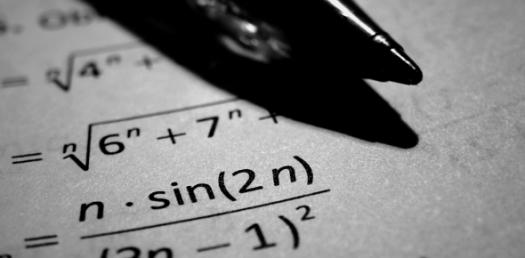# Test Your Math Skills: Can You Pass This Addition Quiz?

48 Questions | Total Attempts: 239SettingsCreate your own QuizBelow is a Test Your Math Skills, Can You Pass This Addition Quiz? Math is one of the most fun subjects to play around with and if you consider yourself a real fan of math and want to see how accurate your calculations are, the quiz below will be a good start. Be sure to give it a try and check out other math quizzes to test our abilities.

• 1.
6+8=
• 2.
7+7=
• 3.
3+9=
• 4.
8+6=
• 5.
3+3=
• 6.
5+3=
• 7.
7+6=
• 8.
5+9=
• 9.
4+7=
• 10.
5+8=
• 11.
7+7=
• 12.
4+6=
• 13.
5+8=
• 14.
5+9=
• 15.
9+4=
• 16.
5+8=
• 17.
9+5=
• 18.
6+5=
• 19.
4+5=
• 20.
6+7=
• 21.
5+9=
• 22.
6+5=
• 23.
6+7=
• 24.
7+5=
• 25.
8+3=
Related TopicsBack to top
×

Wait!
Here's an interesting quiz for you.